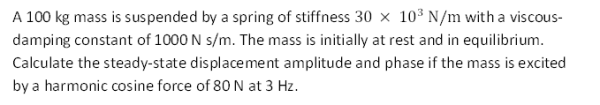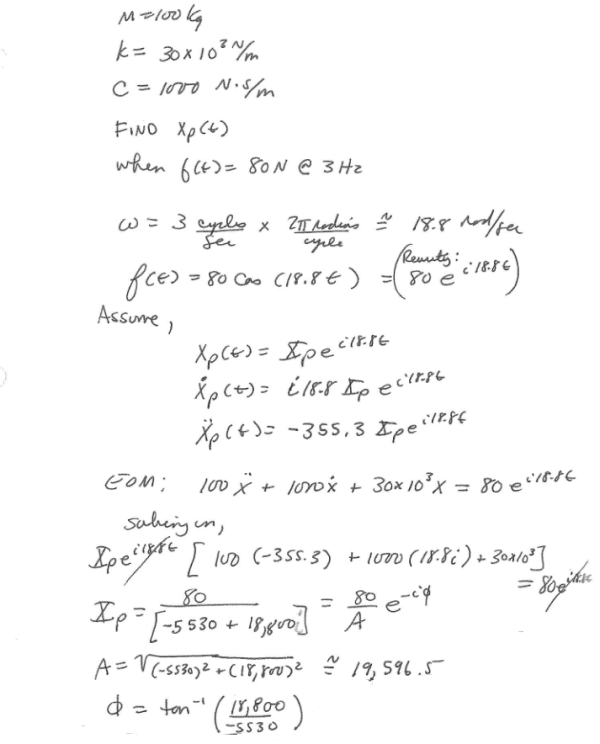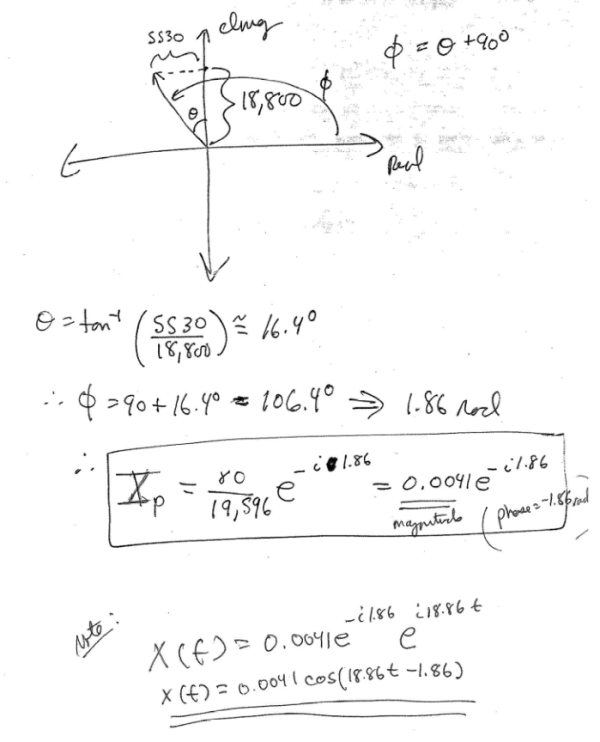A 100 kg mass is suspended by a spring of stiffness 30 x 10^3 N/m with a viscous-damping constant of 1000 N s/m. The mass is initially at rest and in equilibrium. Calculate the steady-state displacement amplitude and phase if the mass is excited by a harmonic cosine force of 80 N at 3 Hz.A 100 kg mass is suspended by a spring of stiffness 30 x 10^3 N/m with a viscous-damping constant of 1000 N s/m. The mass is initially at rest and in equilibrium. Calculate the steady-state displacement amplitude and phase if the mass is excited by a harmonic cosine force of 80 N at 3 Hz.

Vibrations Page 1 vibrations vibrations vibrations vibrations vibrations vibrations vibrations Vibrations Page 2